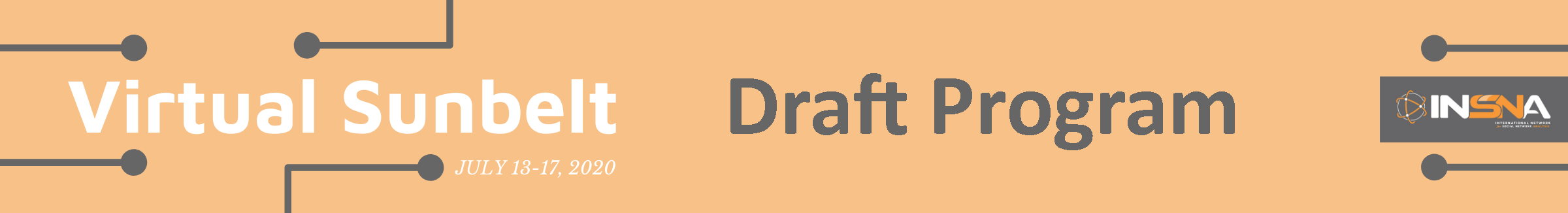## What can we learn about ERGMs?

### Michael Schweinberger, Jonathan Stewart

#### Contact: m.s@rice.edu

Learning ERGMs from network data is challenging, because (1) edge variables are dependent and (2) the number of parameters may increase with the number of population members, e.g., when the model includes node-dependent degree terms as well as triadic terms. Statistical theory shows that, when n independent observations from multivariate distributions with p parameters are available, maximum likelihood estimators are close to the data-generating parameters with high probability when either p is fixed while n increases without bound, or p exceeds n but the model is endowed with additional structure (e.g., the model is sparse in the sense that many parameters are 0) and n increases without bound. In most applications of ERGMs, neither of these two scenarios is applicable. Instead, a single observation of dependent edge variables is observed (when the whole population graph is observed) or a subset of dependent random variables is observed (when a subgraph of the population graph is observed), and the number of parameters p may increase as a function of the number of population members. We derive the most general rates of convergence for maximum likelihood estimators to date, applicable to ERGMs which allow dependence to propagate throughout the population graph and whose number of parameters increases with the number of population members. These rates of convergence are fully non-asymptotic and depend on (1) the dependence among edges, (2) the smoothness of sufficient statistics, and (3) the amount of information contained in the data about the parameters (in the sense of Fisher information). We apply these results to generalized beta-models capturing brokerage in social networks, which allow the propensity to form edges to vary across population members and induce dependence among edges through triadic brokerage terms.

← Schedule